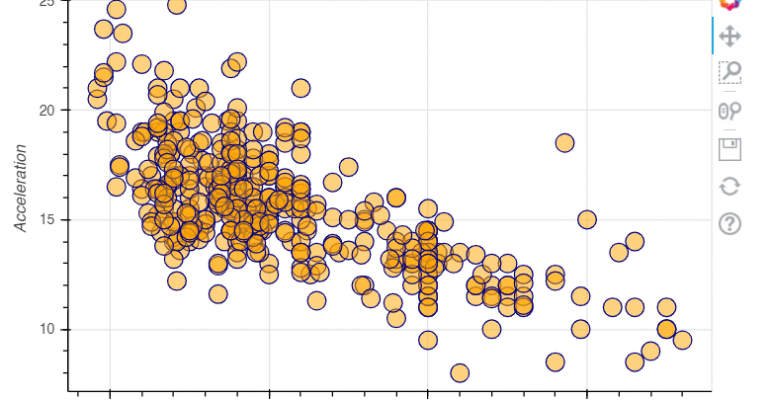Thanks to theidioms.com

### Infosys Tech Scholars Scholarship## Bokeh Scatter Plot – How to plot a scatter plot in Bokeh# Bokeh Scatter Plot – How to plot a scatter plot in Bokeh

Greetings! Some links on this site are affiliate links. That means that, if you choose to make a purchase, The Click Reader may earn a small commission at no extra cost to you. We greatly appreciate your support!

In order to plot a Bokeh scatter plot, you can use a glyph method from Bokeh. There are several glyph markers available in Bokeh such as a cross, asterisk, dot, etc. as shown in the figure below.

The steps to plot a Bokeh scatter plot are as follows:

1. Create a blank figure with necessary arguments: p = figure()
2. Call a glyph method as needed on the figure: p.circle()
3. Show the figure: show(p)

Here is an example of how to create a Bokeh scatter plot using the above steps:

```from bokeh.plotting import *

# Importing sample data
from bokeh.sampledata.autompg import autompg

# See relationships between horse power and acceleration
x = autompg.hp
y = autompg.accel

# Create a blank figure with necessary arguments
p = figure(plot_width=600, plot_height=400,title="Horse Power & Accleration")
p.xaxis.axis_label = 'Horse power'
p.yaxis.axis_label = 'Acceleration'

# Call a glyph method as needed on the figure
p.circle(x,y, size=15, line_color="navy", fill_color="orange", fill_alpha=0.5)

# Show the figure
show(p) ```

This is how to plot a scatter plot in Bokeh.Do you want to learn Python, Data Science, and Machine Learning while getting certified? Here are some best selling Datacamp courses that we recommend you enroll in:

1. Introduction to Python (Free Course) - 1,000,000+ students already enrolled!
2. Introduction to Data Science  in Python- 400,000+ students already enrolled!
3. Introduction to TensorFlow for Deep Learning with Python - 90,000+ students already enrolled!
4. Data Science and Machine Learning Bootcamp with R - 70,000+ students already enrolled!